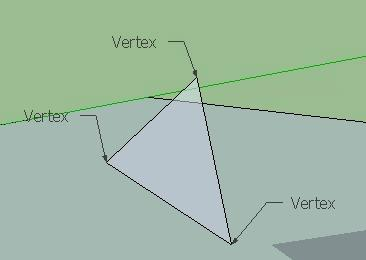To main page | 3dengine.org

# Vertex

Vertex is a point in 3D space, defined by x,y,z coordinates. Couting: 1 vertex, 2 vertices.Shown are three vertices you need to supply to OpenGL in order to draw a triangle.

Vertex coordinates are basically a vector, therefore vector math applies to both of them.

Vertex can have normals, which determine the curvature of the face near this vertex. The normals are usually interpolated unless normal map is present.